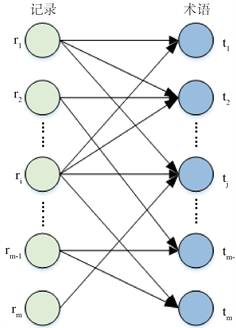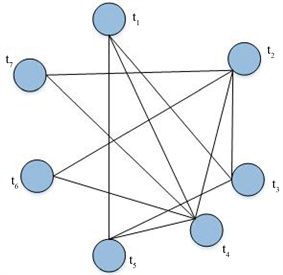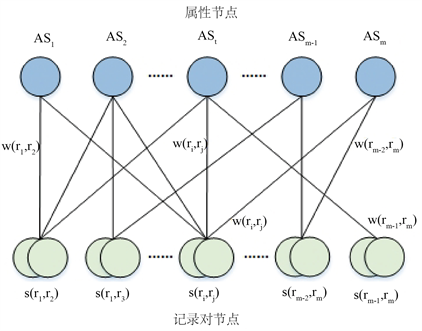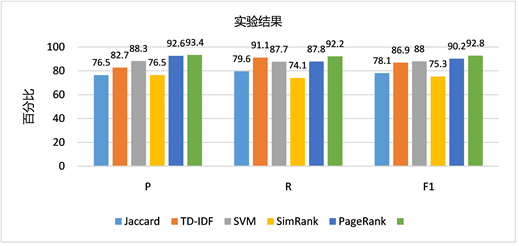# 一种基于属性显著度的实体解析算法An Entity Resolution Algorithm Based on Attribute Salience

DOI: 10.12677/HJDM.2021.112004, PDF, HTML, XML, 下载: 69  浏览: 136

Abstract: Entity resolution (ER) is an important step in data integration and data cleansing. In domain data cleaning and integration, different attributes in an entity usually exhibit different discriminating abilities. Calculating and utilizing the discriminating abilities of attributes can improve the accuracy of record similarity. Current entity resolution methods include record similarity algorithm based on string and algorithm based on machine learning to calculate record similarity, which lacks the im-portance of considering different attributes. Therefore, this paper uses the idea of SimRank and PageRank algorithm and combines the attribute salience obtained by random sampling to propose a similarity algorithm based on attribute salience. Firstly, a weighted attribute record pair bipartite graph is constructed to represent the relationship between attribute and record pair. Secondly, an iterative algorithm for calculating record similarity based on attribute significance is proposed ac-cording to attribute significance combined with graph similarity algorithm. Finally, a record graph is constructed to represent the matching probability between the record pairs (the weight in the bipartite graph), and the improved random walk algorithm is used to estimate the matching probability of the record pairs. Then, the matching probability of record pairs is fed back to the weighted bipartite graph of attribute record pairs, and the weight in the algorithm of calculating record similarity based on attribute salience is modified until convergence. Experi-mental evaluation using real estate data sets shows that the proposed entity resolution algorithm based on attribute salience is more accurate than the mainstream methods.

1. 引言

1) 通过构造一个属性–记录对二部图来建模属性和记录对之间的所属关系。

2) 提出一种基于属性显著度的记录相似性迭代算法，来计算记录对的相似度。

3) 利用记录图来计算记录对之间某一属性的匹配概率。并通过改进的随机游走算法来估算记录图中边的权值(匹配概率)。

4) 在地产领域数据集上进行了实验，验证了算法的有效性。

2. 基于图论的相似度算法

2.1. 基于SimRank相似度算法的相关理论

${S}_{b}\left({r}_{i},{r}_{j}\right)=\frac{{C}_{1}}{|O\left({r}_{i}\right)||O\left({r}_{j}\right)|}{\sum }_{{t}_{i}\in O\left({r}_{i}\right)}{\sum }_{{t}_{j}\in O\left({r}_{j}\right)}{S}_{b}\left({t}_{i},{t}_{j}\right)$ (1)

${S}_{b}\left({t}_{i},{t}_{j}\right)=\frac{{C}_{2}}{|I\left({t}_{i}\right)||I\left({t}_{j}\right)|}{\sum }_{{r}_{i}\in I\left({t}_{i}\right)}{\sum }_{{t}_{j}\in I\left({t}_{j}\right)}{S}_{b}\left({r}_{i},{r}_{j}\right)$ (2)

2.2. 基于PageRank相似度算法的相关理论(a) 二分图(b) 统一图

Figure 1. Graph models in graph-theoretic

$S\left({t}_{i}\right)=\left(1-\phi \right)+\phi \underset{{t}_{i}\in N\left({r}_{i}\right)}{\sum }\frac{s\left({t}_{j}\right)}{|N\left({t}_{i}\right)|}$ (3)

$\phi$ 是阻尼因子通常设置为0.85， $N\left({t}_{i}\right)$ 表示相邻项无向图。两条记录之间基于TW-IDF的文本相似度 ${S}_{u}\left({r}_{i},{r}_{j}\right)$ 由它们共同术语的权重和公式(4)来表示：

${S}_{u}\left({r}_{i},{r}_{j}\right)={\sum }_{t\in {r}_{i}\wedge t\in {r}_{j}}s\left(t\right)\cdot \mathrm{log}\frac{n+1}{df\left(t\right)}$ (4)

3. 基于属性显著度的记录相似度算法

3.1. 基于属性显著度的相似度

$P{S}_{{A}_{m}}=\frac{|\left\{\left({r}_{i},{r}_{j}\right)|{r}_{i}{\approx }_{\sigma }{r}_{j},{A}_{m}\left({r}_{i}\right){\approx }_{{\theta }_{{A}_{m}}}{A}_{m}\left({r}_{j}\right)\right\}|}{|\left\{\left({r}_{i},{r}_{j}\right)|{r}_{i}{\approx }_{\sigma }{r}_{j}\right\}|},0 (5)

$N{S}_{{A}_{m}}=\frac{|\left\{\left({r}_{i},{r}_{j}\right)|{r}_{i}{\ne }_{\tau }{r}_{j},{A}_{m}\left({r}_{i}\right){\approx }_{{\theta }_{{A}_{m}}}{A}_{m}\left({r}_{j}\right)\right\}|}{|\left\{\left({r}_{i},{r}_{j}\right)|{r}_{i}{\ne }_{\tau }{r}_{j},\exists {A}_{n}{A}_{n}\left({r}_{i}\right){\approx }_{{\theta }_{{A}_{n}}}{A}_{n}\left({r}_{j}\right)\right\}|},0 (6)

${S}_{{A}_{m}}=P{S}_{{A}_{m}}-N{S}_{{A}_{m}}$ (7)

${S}_{{A}_{m}}=P{S}_{{A}_{m}}\cdot \left(1-N{S}_{{A}_{m}}\right)$ (8)

$A{S}_{t}={S}_{{A}_{m}}{\sum }_{i\ne j}w\left({r}_{i},{r}_{j}\right)s\left({r}_{i},{r}_{j}\right)s\left({A}_{m}\left({r}_{i}\right),{A}_{m}\left({r}_{j}\right)\right)$ (9)

$S\left({r}_{i},{r}_{j}\right)={\sum }_{i\ne j,t\in {r}_{i}\wedge t\in {r}_{j}}s\left({A}_{m}\left({r}_{i}\right),{A}_{m}\left({r}_{j}\right)\right)\ast A{S}_{t}$ (10)

3.2. 构造属性–记录对之间关系的二部图Figure 2. Bipartite graph for attributes and record-pairs

3.3. 基于二部图的属性显著度的迭代算法

1) 本文用 ${S}_{{A}_{m}}$ 来惩罚属性显著度不高的频繁属性。即公式(8)。

2) 本文构造了一个加权的属性–记录二部图，来估计两个记录指向同一实体的概率 $w\left({r}_{i},{r}_{j}\right)$。即公式(9)。

3) 本文还通过基于记录图的随机游走算法来估计加权二部图的权值(详见第4节)。

1) 在(0, 1)随机初始化 $A{S}_{t}$ (算法第1行)。

2) 如果 ${s}_{t}$ 不收敛，则对记录对 $\left({r}_{i},{r}_{j}\right)$，他的相似度 $s\left({r}_{i},{r}_{j}\right)={\sum }_{t\in {r}_{i}\wedge t\in {r}_{j}}A{S}_{t}$ 进行循环(2~4行)。

3) 将 $s\left({r}_{i},{r}_{j}\right)$ 输入回 $A{S}_{t}$ 循环并标准化 $A{S}_{t}$ (5~7)，如此迭代，直到收敛。

4. 基于随机游走的二部图边权值计算

4.1. 朴素随机游走算法

1) 模拟M次随机游走，其中一半从 ${r}_{i}$ 开始，另一半从 ${r}_{j}$ 开始。

2) 估计 ${r}_{i}$ 到达 ${r}_{j}$ 的概率和 ${r}_{i}$ 到达 ${r}_{j}$ 的概率(第3~7行)。每一次随机游走输出数字1或0，表示冲浪者是否在给定的S步骤内到达目标节点。

3) 然后将成功到达目标的游走的百分比作为匹配概率 $w\left({r}_{i},{r}_{j}\right)$ 的值(第8行)。如果 $\left({r}_{i},{r}_{j}\right)$ 是匹配对，那么到达概率应接近1。

4.2. 构造记录图

4.3. 改进的随机游走算法

$w\left({r}_{i},{r}_{j}\right)=\frac{{\left(1+\beta \right)}^{\alpha }s{\left({r}_{i},{r}_{j}\right)}^{\alpha }}{{\sum }_{{r}_{j}\in O\left({r}_{i}\right)}s{\left({r}_{i},{r}_{j}\right)}^{\alpha }}$ (11)

5. 实验

5.1. 数据集

5.2. 方法比较

5.3. 实验结果及分析Figure 3. Experimental result

6. 结论

  Christophides, V., Efthymiou, V. and Stefanidis, K. (2015) Entity Resolution in the Web of Data: Synthesis Lectures on the Semantic Web: Theory and Technology. Morgan & Claypool Publishers. https://doi.org/10.2200/S00655ED1V01Y201507WBE013  韦海浪, 李贵, 李征宇, 韩子扬, 曹科研. 半结构化实体解析算法[J]. 数据挖掘, 2020, 10(1): 1-15. https://doi.org/10.12677/HJDM.2020.101001  Kenig, B. and Gal, A. (2013) MFIBlocks: An Effective Block-ing Algorithm for Entity Resolution. Information Systems, 38, 908-926. https://doi.org/10.1016/j.is.2012.11.008  Kolb, L., Thor, A. and Rahm, E. (2012) Dedoop: Efficient Deduplica-tion with Hadoop. Proceedings of the VLDB Endowment, 5, 1878-1881. https://doi.org/10.14778/2367502.2367527  高广尚, 张智雄. 关于实体解析基本方法的研究和述评[J]. 数据分析与知识发现, 2019, 3(5): 27-40.  Bilenko, M. and Mooney, R.J. (2003) Adaptive Duplicate Detection Using Learnable String Similarity Measures. Proceedings of the 9th ACM SIGKDD International Conference on Knowledge Discovery and Data Mining, Washington DC, August 2003, 39-48. https://doi.org/10.1145/956750.956759  Cohen, W.W. (2000) Data Integration Using Similarity Joins and a Word-Based Information Representation Language. Information Systems, 18, 288-321. https://doi.org/10.1145/352595.352598  Ristad, E. S. and Yianilos, P.N. (1998) Learning String-Edit Distance. IEEE Transactions on Pattern Analysis and Machine Intelligence, 20, 522-532. https://doi.org/10.1109/34.682181  Bilenko, M. and Mooney, R.J. (2002) Learning to Combine Trained Dis-tance Metrics for Duplicate Detection in Databases. TechRep AI, 02-296.  Tejada, S., Knoblock, C.A. and Minton, S. (2001) Learning Object Identification Rules for Information Integration. Information Systems, 26, 607-633. https://doi.org/10.1016/S0306-4379(01)00042-4  Cohen, W.W. and Richman, J. (2002) Learning to Match and Cluster Large High-Dimensional Data Sets for Data Integration. Proceedings of the 8th ACM SIGKDD International Conference on Knowledge Discovery and Data Mining, July 2002, 475-480. https://doi.org/10.1145/775047.775116  Ravikumar, P.D. and Cohen, W.W. (2004) A Hierarchical Graphical Model for Record Linkage. Proceedings of the 20th Conference on Uncertainty in Artificial Intelligence, 454-461.  张晓辉, 蒋海华, 邸瑞华. 基于属性权重的链接数据共指关系构建[J]. 计算机科学, 2013, 40(2): 40-43.  强保花, 吴忠福. 基于属性信息熵的实体匹配方法研究[J]. 计算机工程, 2005, 31(21): 31-33.  Brin, S. and Page, L. (2002) The Anatomy of a Large-Scale Hypertextual web Search Engine. Computer Networks and ISDN Systems, 30, 107-117. https://doi.org/10.1016/S0169-7552(98)00110-X  Jeh, G. and Widom, J. (2002) Simrank: A Meas-ure of Structural-Context Similarity. KDD’02: Proceedings of the 8th ACM SIGKDD International Conference on Knowledge Discovery and Data Mining, July 2002, 538-543. https://doi.org/10.1145/775047.775126  Zhang, D., Guo, L., He, X., et al. (2018) A Graph-Theoretic Fusion Framework for Unsupervised Entity Resolution. 2018 IEEE 34th International Conference on Data Engineering (ICDE), Paris, 16-19 April 2018, 713-724. https://doi.org/10.1109/ICDE.2018.00070